# The diffusion distance

Let $$G=(V,E)$$ be a single-layer unweighted and undirected network. Let $$\mathbf{A}$$ be its adjacency matrix, i.e. $$|V| = N$$, the nodes in $$V$$ are labelled as $$i \in 1, \dots, N$$ and $$\mathbf{A}$$ is an $$N \times N$$ symmetric matrix such that $$A_{ij} = A_{ji}= 1$$ if and only if $$\{i, j\} \in E$$ and $$A_{ij} = A_{ji}= 0$$ otherwise. The degree of vertex $$i$$ is the number of its adjacent nodes, i.e. $$k_i = \sum_{j = 1}^{N} A_{ij} = \left(\mathbf{A} \mathbf{1}\right)_i$$, and we collect the degrees of all vertices into the diagonal matrix $$\mathbf{D}$$. Let us also assume that there are no isolated nodes, i.e. $$k_i > 0$$ for all $$i \in 1, \dots, N$$ or, if there are isolated nodes, we use the convention $$(k_i)^{-1} = 0$$ if $$k_i = 0$$.

The matrix $$\mathbf{T} = \mathbf{D}^{-1} \mathbf{A}$$ can be seen as the transition matrix of a random walk on the network $$G$$, a discrete-time Markov chain on the set of vertices $$V$$, with transition probabilities given by the connectivity of $$G$$. $$T_{ij} = \frac{A_{ij}}{k_i}$$ is the probability of going from node $$i$$ to node $$j$$ and it is different from 0 only if there is an edge connecting $$i$$ and $$j$$. Furthermore, from $$i$$ the random walker has equal probability of transitioning to each of its neighbours (adjacent nodes). Observe that $$\mathbf{T}^k$$ is the k-step transition probability matrix, which is however not immediate to compute, since $$\mathbf{T}$$ is, in general, not symmetric and $$N$$ can be very large.

From this discrete-time random walk we can also define its versiona continuous-time Markov chain with exponential holding times with rate $$\lambda = 1$$ and jumping probabilities given by $$\mathbf{T}$$ (Norris 1998; Masuda, Porter, and Lambiotte 2017). In particular the continuous-time process is generated by the $$Q-$$matrix $$-(\mathbf{I}- \mathbf{T})$$, where $$\mathbf{I}- \mathbf{T} = \mathbf{\tilde{L}}$$ corresponds to the (random walk) normalised Laplacian (F. R. K. Chung 1997) of $$G$$, and the dynamics is controlled by the forward (or master) equation $\begin{equation} \frac{d}{d\tau}\mathbf{p}(\tau) = - \mathbf{p}(\tau) \mathbf{\tilde{L}}. \end{equation}$

Given the initial condition $$\mathbf{p(\tau = 0)} = \mathbf{e}_i$$, the canonical vector with components $$e_j = 0$$ for all $$j\neq i$$ and $$e_{i} = 1$$, which corresponds to the deterministic initial condition with the random walker starting in node $$i$$, the solution of the master equation at time $$\tau > 0$$ is $\begin{equation} p_{ij} = \left(e^{-\tau \tilde{\mathbf{L}}}\right)_{ij} \end{equation}$ and can be equivalently seen as: * the transition probability from node $$i$$ to node $$j$$ in the time interval $$[0, \tau]$$, or * the probability of fining the random walker (i.e. the process) in node (state) $$j$$ at time $$\tau$$ given that it started in node $$i$$ at time 0.

Let us call $$\mathbf{P}(\tau) = e^{-\tau \tilde{\mathbf{L}}}$$. $$\mathbf{P}(\tau)$$ is a stochastic matrix and is given by $\begin{equation} e^{-\tau \tilde{\mathbf{L}}} = \sum_{h = 0}^{+\infty} \frac{(-\tau \tilde{\mathbf{L}})^h}{h!}. \end{equation}$ Again here we have powers of a non-symmetric matrix. However, the normalised Laplacian can be written as $$\tilde{\mathbf{L}} = D^{-\frac12}\mathcal{L}^{\text{sym}}D^{\frac12}$$, with $$\mathcal{L}^{\text{sym}} = D^{-\frac12} (D - A) D^{-\frac12}$$ being the symmetric normalised Laplacian, which has a spectrum of real, non-negative eigenvalues, and whose eigenvectors can be chosen to form an orthogonal matrix $$\mathbf{U}$$ (F. R. K. Chung 1997), so that $$\mathcal{L}^{\text{sym}} = \mathbf{U} \boldsymbol{\Lambda} \mathbf{U}^T$$. It follows that $$\tilde{\mathbf{L}} = D^{-\frac12} \left( \mathbf{U} \boldsymbol{\Lambda} \mathbf{U}^T \right) D^{\frac12}$$ and the evaluation of $$e^{-t \tilde{\mathbf{L}}}$$ reduces to a diagonalisation of the symmetric normalised Laplacian and to the exponentiation of its spectrum, i.e. $$e^{-t \tilde{\mathbf{L}}} = \left(D^{-\frac12} \mathbf{U} \right) e^{-t\boldsymbol{\Lambda}} \left(\mathbf{U}^T D^{\frac12}\right)$$.

Let us denote by $$\mathbf{p}(\tau|i) = \left(e^{-t \tilde{\mathbf{L}}}\right)_{i\cdot}$$ the probability vector corresponding to the $$i-$$th row of the exponential matrix $$e^{-t \tilde{\mathbf{L}}} = \mathbf{P}(t)$$, which gives the posterior probability distribution of finding the random walker across the network at time $$t$$ given that the walk started in node $$i$$ with probability 1. The Euclidean distance between two rows of matrix $$\mathbf{P}(t)$$ and hence, the distance between two posterior probability distributions corresponding to different starting nodes, induced a distance between the nodes of the network, called the (De Domenico 2017):

$\begin{equation} D_{\tau}(i, j) = \lVert \mathbf{p}(\tau|i) - \mathbf{p}(\tau|j) \rVert_2. \end{equation}$

To be precise this construction yields a family of diffusion distances $$\left(D_{\tau}(i, j)\right)_{t>0}$$, because of the dependence of $$D_{\tau}$$ on time $$t$$. As shown in (Coifman and Lafon 2006; Lambiotte, Delvenne, and Barahona 2014; De Domenico 2017) $$t$$ serves as a resolution parameter. Intuitively, for increasing time $$t$$ the Markov chain (or the random walker) can explore larger parts of the network and this is particularly evident if we look at the discrete-time jump chain $$\mathbf{p}(n + 1) = \mathbf{p}(n) \mathbf{T} = \mathbf{p}(0) \mathbf{T}^n = \mathbf{p}(0) \left(\mathbf{D}^{-1}\mathbf{A}\right)^n$$. It is known that the $$ij-$$th entry of the $$n-$$th power of the adjacency matrix, $$\left(\mathbf{A}^n\right)_{ij}$$, counts the walks of length $$n$$ starting in $$i$$ and ending in $$j$$. Integrating $$D_{\tau}$$ over time, to get rid of the dependence on $$\tau$$, yields the persistent functional patterns in networks.

The extensions of this distance to weighted, directed or even more complicated networks, such as multilayers is easily integrated as soon as we can define the transition rules from node to node. For weighted directed networks we just need to replace the dyadic adjacency matrix with its weighted counterpart $$\mathbf{W}$$ and a node’s degree with its out-going strength. In (Bertagnolli and De Domenico 2021) for instance, the diffusion distance has been generalised not only to multiplex and interconnected systems, but also to different types of random walk dynamicse.g. maximal-entropy or PageRank random walkstaking place on the same networked system.

The diffusion distances are bounded in $$[0, \sqrt{2}]$$ since $$\lVert \mathbf{p}(\tau|i) \rVert_2 \leq \lVert \mathbf{p}(\tau|i) \rVert_1 = 1$$ for all nodes $$i \in V$$. Furthermore the average squared diffusion distance (ASDD) $\begin{equation} \mathcal{D}^2_{\tau} = \frac{1}{2 N^2} \sum_{i, j = 1}^N D^2_{\tau}(i, j) \end{equation}$ is equal to the sum of the (biased) sample variances of the random vector $$\mathbf{p}(\tau|i)$$, $$\sum\limits_{i=1}^N \sum\limits_{l=1}^N \left(p_{il} - \bar{p}_i\right)^2$$ and, thus, it is a measure of the dispersion of the network. The proof follows from the known equivalence $s_{X}^{2} = \text{cov}(X, X) = \frac{1}{2 N^2} \sum_{i=1}^{N} \sum_{j=1}^{N} \left(x_{i}-x_{j}\right)^{2}$ for a sample from a random variable $$X$$.

# Using the diffudist package

The main function in the diffudist is get_distance_matrix(), or its shorter alias get_DDM(), which returns the distances $$D_{\tau}(i, j)$$ for all nodes $$i,j$$ in the network at some time $$\tau\geq 0$$, as presented in the previous section. Its arguments are

get_distance_matrix(g, tau, type = "Normalized Laplacian", weights = NULL,
as_dist = FALSE, verbose = TRUE)

where g is the network (in the igraph format), whose nodes you want to compute distances for; tau is the parameter $$\tau$$ in the master equation controlling the diffusion time. type refers to the type of dynamics used for the mapping from nodes to points in the diffusion space. By default it is set to “Normalized Laplacian,” $$\mathbf{\tilde{L}} = \mathbf{I} - \mathbf{D}^{-1} \mathbf{A}$$, the generator of the classical (node-centric) continuous-time random walk as described in Section . Other types of random walk dynamics generators are available and will be described at the end of this section. The weights parameter allows to switch from a weighted to an unweighted network. Mind that, similarly to the igraph package (Csardi and Nepusz 2006), if weights = NULL and the network g has and edge attribute called “weight,” then this is used automatically. Finally, as_dist gives the possibility to control the class of the returned object (either a matrix or a “dist” object) and only if verbose is set to TRUE messages about the progress and warnings are shown.

## The classical random walk and its induced diffusion distances

To illustrate the usage of the package diffudist let us start with a small well-known network: Zachary’s karate club network (Zachary 1977). After loading the packages igraph, for manipulating networks, and our diffudist, we download the weighted adjacency matrix of the karate club network1 and build the network karate:

library(igraph)
library(diffudist)
igraph_options(vertex.frame.color = "white", vertex.color = "#00B4A6")
# dataset
skip = 41, sep = " ", header = FALSE)
A <- as.matrix(df[, -1])
colnames(A) <- rownames(A) <- c("Mr Hi", paste("Actor", 2:33), "John A")
karate <- graph_from_adjacency_matrix(A, mode = "undirected", weighted = TRUE)
V(karate)$name <- c("H", 2:33, "A") V(karate)$Faction <- 1
V(karate)$Faction[c(9, 10, 15, 16, 19, 21, 23:34)] <- 2 V(karate)$color <- viridis::viridis(3, direction = -1)[V(karate)$Faction] Plot the network: plot(karate, edge.width = E(karate)$weight)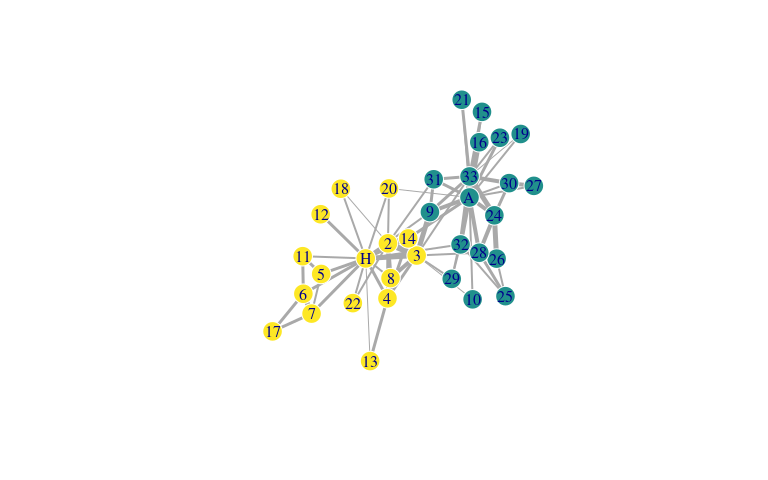Figure 1 Zachary’s karate club network. The node colour represents the ‘faction’ the corresponding Actor belongs to after the club splits. Nodes labelled as ‘A’ and ‘H’ correspond respectively to John A., the club administrator, and Mr. Hi, the instructor.

The karate network has $$N=34$$ nodes and 78 edges, different vertex attributes and the weight attribute of its edges. The nodes in Zachary’s karate club network are members of a university karate club, called Actors in the social networks jargon (see head(V(karate)$name)) and the weighted links connecting them encode interactions outside the club during three years, which were documented by Zachary. The network is well-known especially as a community detection benchmark, seeing as how Zachary was able (and lucky) to witness and document a conflict that arose during the study and eventually led to the splitting of the club in two “Factions” centred around the instructor (Mr. Hi) and the club administrator (John A.). karate_unw_ddm_list <- lapply(1:10, function(tau) { get_distance_matrix(karate, tau = tau, weights = NA, verbose = FALSE) }) karate_unw_ddm_plots <- lapply(c(1, 2, 4, 8), function(i) { ddm <- karate_unw_ddm_list[[i]] plot_distance_matrix(ddm, cex = 1.3, title = bquote(tau==.(i))) }) invisible(lapply(karate_unw_ddm_plots, show))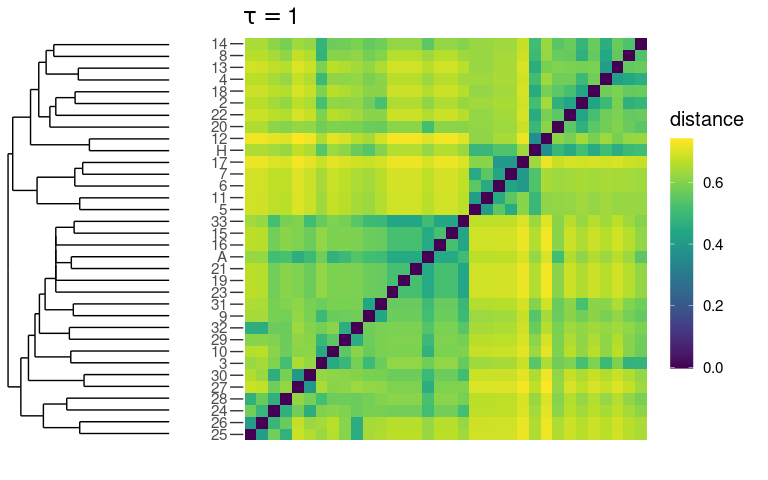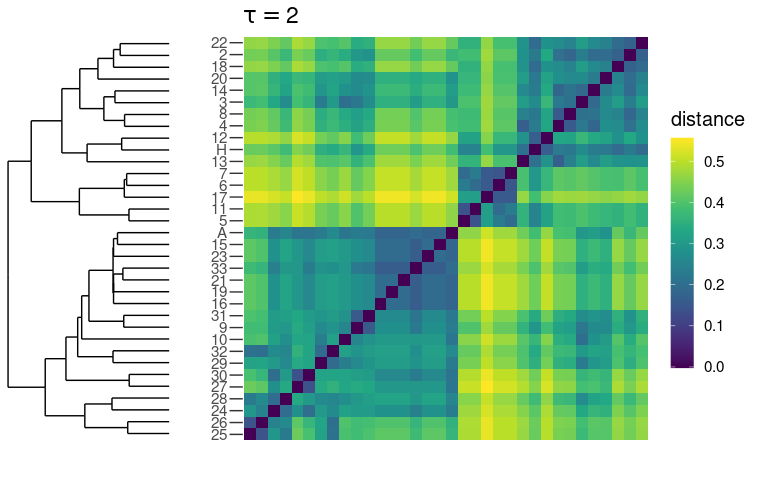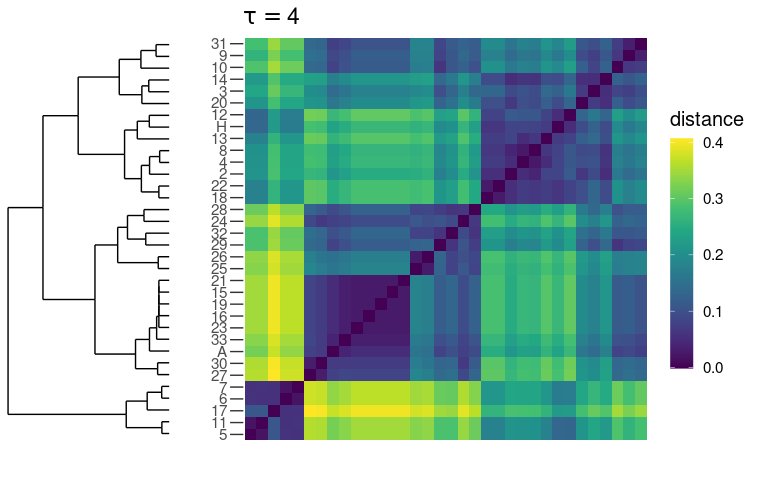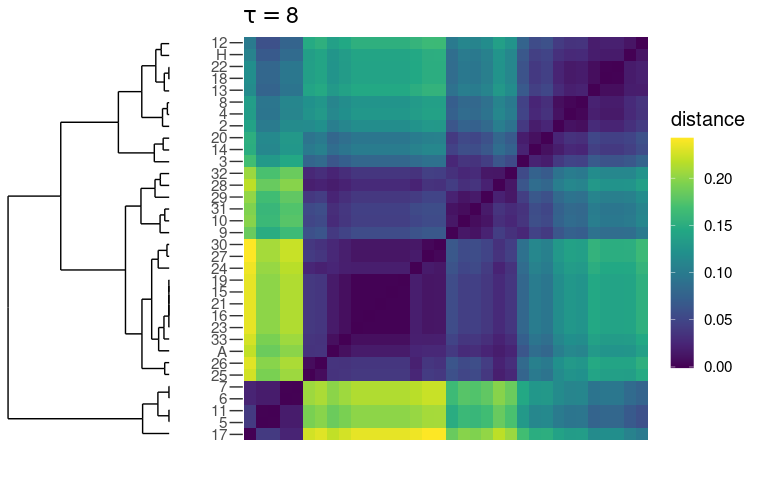Figure 2 Diffusion distances of the unweighted karate club network at different diffusion times and w.r.t. the classical random walk (CRW). As one can see in Fig.2, at small time scales $$\tau = 1, 2$$, plots a) and b), there are two main blocks, while already at $$\tau = 4$$ a small cluster, consisting of Actors 5, 6, 7, 11 and 17, emerges These nodes form almost a clique and are connected only to Mr. Hi and no other node in the network. They hence form a distinct functional cluster at larger resolutions. This “issue” is well-known among network scientists and, indeed, several algorithms for community detection actually find four clusters instead of two (Fortunato 2010), although the two module partition is more stable (Arenas, Fernández, and Gómez 2008). Another common misclassification (Fortunato 2010) involves Actors 3, 9 and 10. The first follows Mr. Hi after the fission, while the other two follow John A. At $$\tau = 1$$ all three nodes fall in the same clustered with John A., while at $$\tau = 2$$ Actor 3 becomes closer to Mr. Hi in agreement with the real post-conflict configuration. This misclassification does not happen when edge weights, the strength of the ties between the club members, are taken into account, as you can see in Fig.3. karate_w_ddm_list <- lapply(1:10, function(tau) { get_distance_matrix(karate, tau = tau, verbose = FALSE) }) res_clu <- hclust(d = as.dist(karate_w_ddm_list[])) memb <- cutree(res_clu, k = 2) V(karate)$cluster <- cutree(res_clu, k = 2)
plot(karate, vertex.shape = c("circle", "square")[V(karate)\$cluster])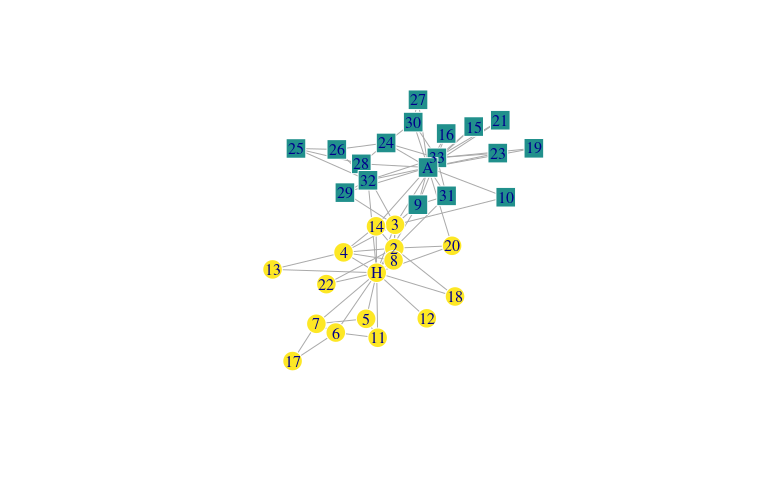Figure 3 Community detection of the weighted karate club network through hierarchical clustering of diffusion distances at $$\tau = 1$$. As in Fig.1 the colour of nodes depends on their faction, while their shape corresponds to the cluster assigned by the hierarchical clustering hclust on the diffusion distances $$D_{\tau = 1}$$.

Also, if we look at the distance matrices in Fig.4 we find a persistent organisation of nodes in two main modules. Finally note that, overall, the pairwise distances become smaller as $$\tau$$ increases2, nevertheless the intra-community distances shrink faster than the inter-communities distances, as found in (De Domenico 2017).

karate_w_ddm_plots <- lapply(c(1, 2, 4, 8), function(i) {
ddm <- karate_w_ddm_list[[i]]
plot_distance_matrix(ddm, cex = 1.2, title = bquote(tau==.(i)))
})
invisible(lapply(karate_w_ddm_plots, show))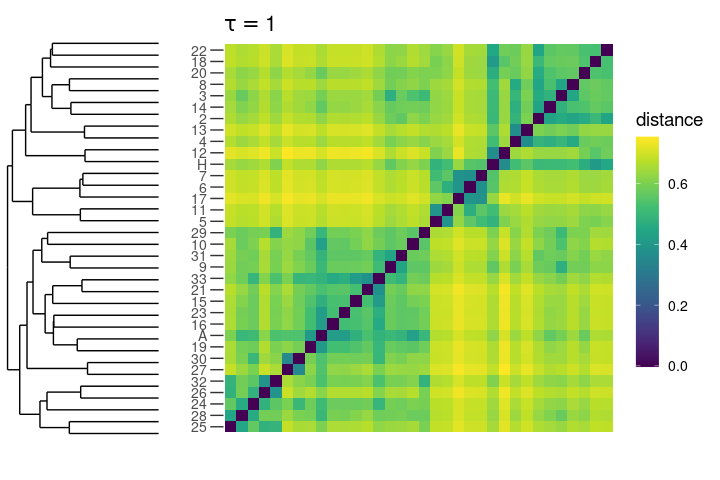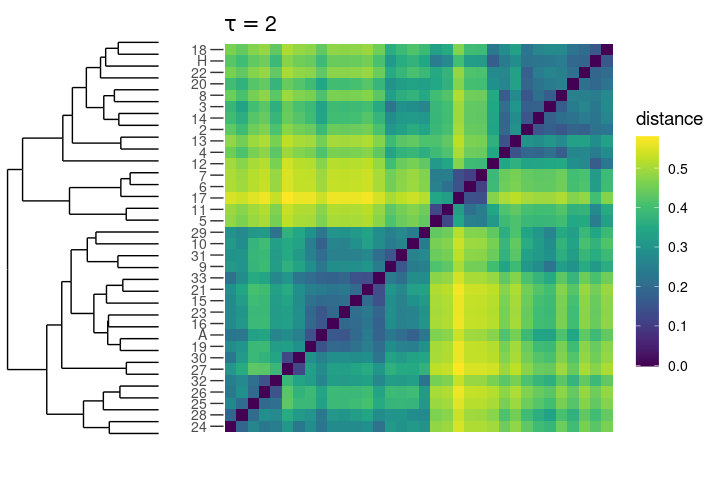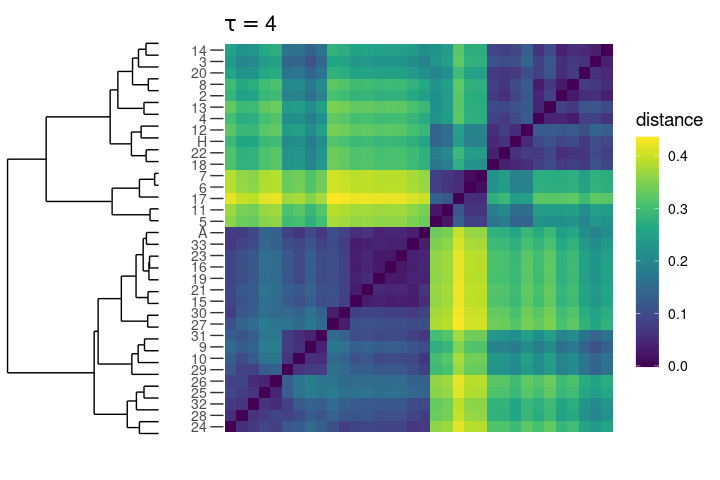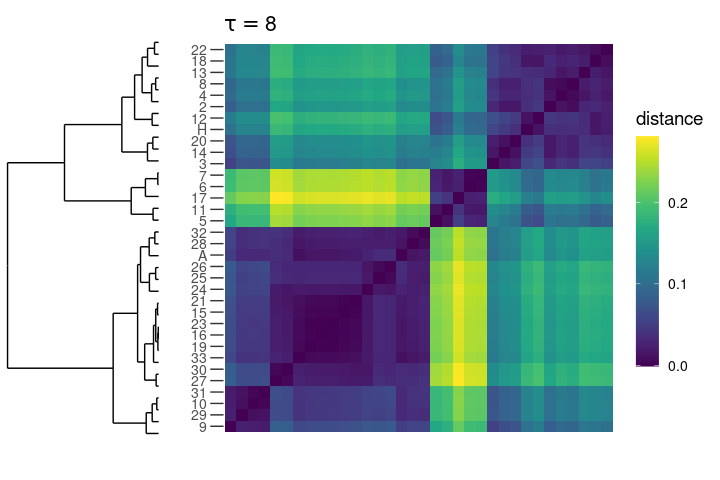Figure 4 Diffusion distances of the weighted karate club network. As in Fig.2, but accounting also for interactions strenghts (i.e. edge weights). Observe that in this case there are no misclassifications and the (main) two-module partition is stable over time.

In order to compare diffusion distances at different times or between different networks, one can normalise the distance matrix $$\mathbf{D}_{\tau}$$ over the maximum, i.e. $$\mathbf{\hat{D}}_{\tau} = \frac{\mathbf{D}_{\tau}}{\max{\mathbf{D}_{\tau}}}$$, as shown in Fig. 5 for the average distance matricesobtained calling the function get_mean_distance_matrix() on the list of diffusion distance matrices at different values of $$\tau$$of the unweighted and weighted karate club network. As mentioned in Section , averaging diffusion distances over time yields the persistent diffusion geometry of the network, which is very similar for both its versions (weighted and not).

karate_unw_ddm_avg <- get_mean_distance_matrix(karate_unw_ddm_list)
karate_w_ddm_avg <- get_mean_distance_matrix(karate_w_ddm_list)
# then divide by max() and plot as before (but with  show_dendro = FALSE)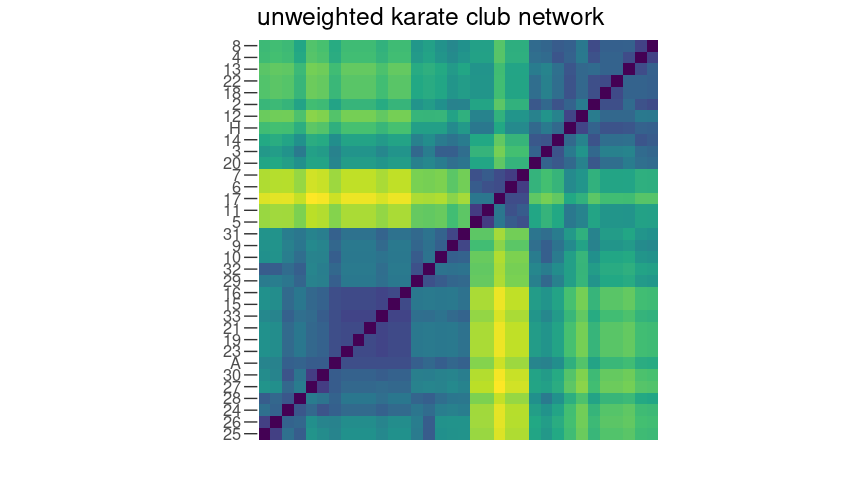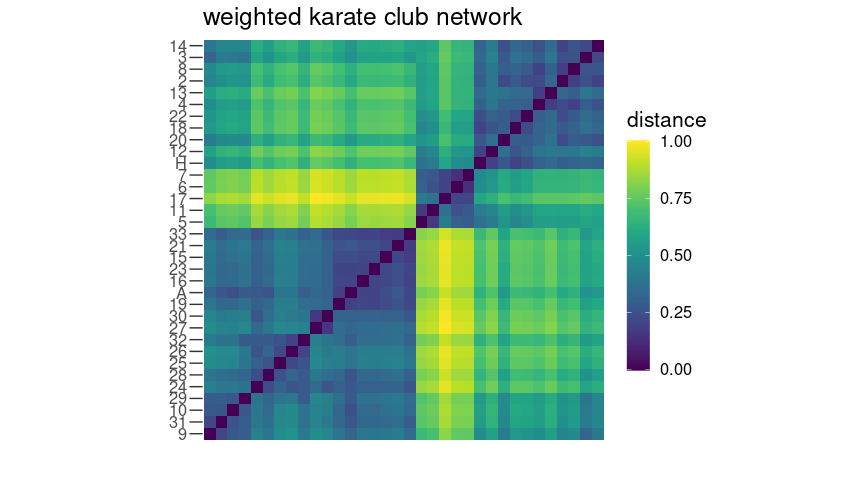Figure 5 Comparison of the normalised average diffusion distance matrices for the unweighted/weighted karate club network.

## Other random walk dynamics

Until now, we have studied the functional shape of a network revealed by the diffusion distance based on the classical random walk (CRW), which is characterised by the transition matrix $$\mathbf{T} = \mathbf{D}^{-1} \mathbf{A}$$ and its corresponding normalised Laplacian. There are, although, other possibilities; a few other types of random walk dynamics are already implemented in the diffudist and, in particular:

• the unnormalised Laplacian $$\mathbf{L} = \mathbf{D} - \mathbf{A}$$, generating an edge-centric continuous-time random walk (Masuda, Porter, and Lambiotte 2017);
• the quantum laplacian, which is equivalent to the symmetric normalised Laplacian $$\mathcal{L}^{\text{sym}}$$ and generates a valid quantum (random) walk $$P(t) = e^{-i \mathcal{L}^{\text{sym}} t}$$ with $$i$$ being the imaginary unit (Biamonte, Faccin, and De Domenico 2019);
• the maximal-entropy random walk (MERW) Laplacian $$\mathbf{I} - \mathbf{T}^{\text{MERW}}$$, where $$T_{ij}^{\text{MERW}} = \frac{A_{ij}}{\lambda}\frac{\psi_i}{\psi_j}$$ with $$\lambda$$ being the eigenvalue of the adjacency matrix and $$\boldsymbol{\psi} \mathbf{A} = \lambda \boldsymbol{\psi}$$ is the corresponding normalised eigenvector (Burda et al. 2009).

Additionally, one can also provide a generic (valid) transition matrix $$T$$ (also called $$\Pi$$ in the literature and called Pi in the code) and use get_distance_matrix_from_T(Pi, tau, verbose = TRUE) to obtain the mutual diffusion distances w.r.t. the custom random walk dynamic. Let’s look, for instance at the PageRank random walk (PRRW) Laplacian $$\mathbf{I} - \mathbf{T}^{\text{PRRW}}$$ (Brin and Page 1998; F. Chung and Zhao 2010): this random walk is characterized by a teleportation parameter $$\alpha \in [0, 1)$$ which, at each step, enables the random walker to jump to any node (chosen uniformly at random) in the network. In particular the walker can reach (i) with probability $$1 - \alpha$$ any node in the network and (ii) with probability $$\alpha$$ one of its adjacent nodes. The master equation for the discrete-time PRRW reads $p_{i}(n+1) = \alpha \sum_{j=1}^{N} p_{j}(n) T^{\text{CRW}}_{j i} + (1-\alpha) u_{i}$ where $$u_{i}$$ is a vector satisfying $$\sum_{i=1}^N u_{i} = 1$$, usually a uniform probability vector $$\frac1N \mathbf{1}$$, where is a (row) vector of all ones. The transition matrix is then given by $\mathbf{T}^{\text{PRRW}} = \alpha \mathbf{D}^{-1} \mathbf{A} + (1 - \alpha) \frac1N \mathbf{1}^T \mathbf{1}$

alpha <- .85
N <- gorder(karate)
D_inv_karate <- diag(1 / strength(karate))
W_karate <- as_adjacency_matrix(karate, sparse = FALSE, attr = "weight")
T_prrw <- alpha * D_inv_karate %*% W_karate + (1 - alpha) / N
# its corresponding Laplacian would be:
# L_prrw <- diag(rep(1, N)) - T_prrw
ddm_prrw <- get_distance_matrix_from_T(Pi = T_prrw, tau = 2, verbose = FALSE)

Observe that in this example we are giving the random walker the possibility to teleport to itself. If you want to exclude this possibility, instead of having $$\alpha \frac1N \mathbf{1}^T \mathbf{1}$$ chose $$\frac{1}{N-1} (\mathbf{1}^T \mathbf{1} - \mathbf{I})$$.

plot_distance_matrix(ddm_prrw, cex = 1.2)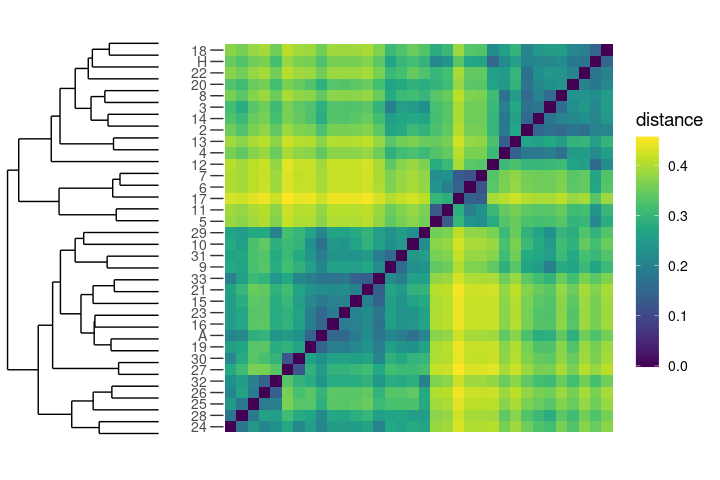Distance matrix of the karate club network at $$\tau=2$$ for the PRRW.

## Diffusion distances of large networks

To and large ($$N>1000$$) networks correspond large (usually sparse) matrices and this increases the computational cost of evaluating $$e^{-t\mathbf{L}}$$, for some network Laplacian $$\mathbf{L}$$3, which is approximately $$O(N^3)$$ (Moler and Van Loan 2003). By default the function get_distance_matrix() computes the required type of Laplacian and passes it to the function expm of the homonymous expm (Goulet et al. 2021), which is written in and computes the (Padé-Approximation of the) matrix exponential with the “Scaling & Squaring” method. Alternatively, one can choose to compute the eigendecomposition of the Laplacian, e.g. through get_spectral_decomp() for the normalised Laplacian $$\mathbf{\tilde{L}} = \mathbf{I} - \mathbf{D}^{-1}\mathbf{A}$$, and then evaluating the distances using get_ddm_from_eigendec().

1. The karate network is also available as an unweighted network in igraph (Csardi and Nepusz 2006) make_graph("Zachary") and as a weighted network in the data package igraphdata (Csardi 2015).↩︎

2. The karate club network is connected and non-bipartite, hence the random walk on it is ergodic and the process converges to the invariant/stationary distribution (F. R. K. Chung 1997).↩︎

3. The complexity of the actual computation of diffusion distances reduces to that of the matrix exponential, since the Euclidean distances are computed efficiently using the dist() function of the package stats or, if available, its parallelised version Dist() of parallelDist (Eckert 2018)↩︎